Tangent sheaf

The sheaf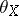on an algebraic variety or schemeover a field, whose sections over an open affine subspace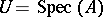are the-modules of-derivations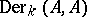of the ring. An equivalent definition is thatbe the sheaf of homomorphisms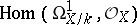of the sheaf of differentials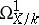into the structure sheaf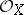(see Derivations, module of).
For any rational-point, the stalk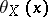of the sheafis identical to the Zariski tangent spacetoat, that is, to the vector-space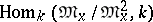, where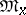is the maximal ideal of the local ring. Instead of the tangent sheafone can use the sheaf of germs of sections of the vector bundle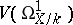dual to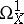(or the tangent bundle to). In the case whenis a smooth connected-scheme,is a locally free sheaf onof rank equal to the dimension of.